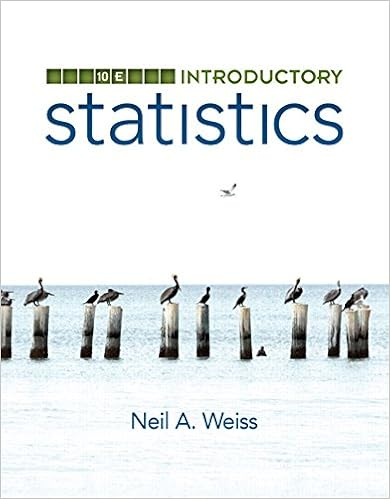# Distance and Midpoint - Geometry Writing Assignment:...

• Homework Help
• 4

This preview shows page 1 - 4 out of 4 pages.

The preview shows page 3 - 4 out of 4 pages.
GeometryWriting Assignment: Distance and Midpoint FormulasEach problem is worth 5 pointsTotal Points: 50Distance Formula1.Find the distance between the points (-4, 6) and (8, -12).Round your solution to 2 decimal points.2.Find the perimeter of the triangle below. Round your solution to 2 decimal points.
##### We have textbook solutions for you!The document you are viewing contains questions related to this textbook.
Chapter 9 / Exercise 21
Discrete Mathematics With Applications
EppExpert VerifiedBrowse all Textbook Solutions
3.Find the length of diagonal XU in the hexagon below. Round your solution to 2 decimal points.4. Find the distance between the two points. Round your solution to 2 decimal points.
5.
End of preview. Want to read all 4 pages?

Course Hero member to access this document

Term
Spring
Professor
Tina Kushner
Tags
Distance, midpoint formulas
##### We have textbook solutions for you!
The document you are viewing contains questions related to this textbook.The document you are viewing contains questions related to this textbook.
Chapter 9 / Exercise 21
Discrete Mathematics With Applications
EppExpert Verified
•••